Next: The 1-d wave equation Up: The wave equation Previous: The Crank-Nicholson scheme

## Upwind differencing

We might be forgiven for concluding that the Crank-Nicholson scheme represents an efficient and accurate general purpose numerical method for solving the advection equation. This is indeed the case, provided we restrict ourselves to fairly smooth wave-forms. Unfortunately, the Crank-Nicholson scheme does a very poor job at advecting wave-forms with sharp leading or trailing edges. This is illustrated in Fig. 78, which shows a calculation in which the Crank-Nicholson scheme is used to advect a square wave-pulse. It can be seen that spurious oscillations are generated at both the leading and trailing edges of the wave-form. It turns out that all central difference schemes for solving the advection equation suffer from a similar problem.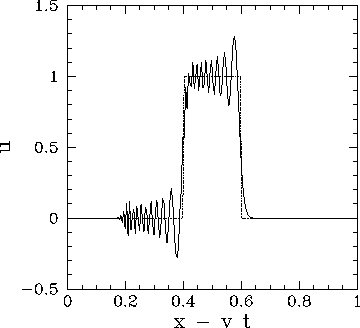The only known way to suppress spurious oscillations at the leading and trailing edges of a sharp wave-form is to adopt a so-called upwind differencing scheme. In such a scheme, the spatial differences are skewed in the upwind'' direction: i.e., the direction from which the advecting flow emanates. Thus, the upwind version of the simple explicit differencing scheme (236) is written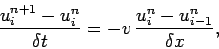(248)

or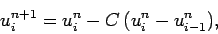(249)

Note that this scheme is only first-order in space, whereas every other scheme we have discussed has been second-order. A von Neumann stability analysis of the above scheme yields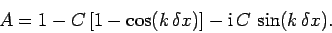(250)

Note that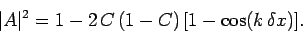(251)

It follows that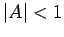for allprovided that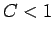. Thus, the upwind differencing scheme is stable provided that the CFL condition is satisfied. Fig. 79 shows a calculation in which the above scheme is used to advect a square wave-pulse. There are now no spurious oscillations generated at the sharp edges of the wave-form. On the other hand, the wave-form shows evidence of dispersion. Indeed, the upwind differencing scheme suffers from the same type of spurious dispersion problem as the Lax scheme. Unfortunately, there is no known differencing scheme which is both non-dispersive and capable of dealing well with sharp wave-fronts. In fact, sophisticated codes which solve the advection (or wave) equation generally employ an upwind scheme in regions close to sharp wave-fronts, or shocks, and a more accurate non-dispersive scheme elsewhere.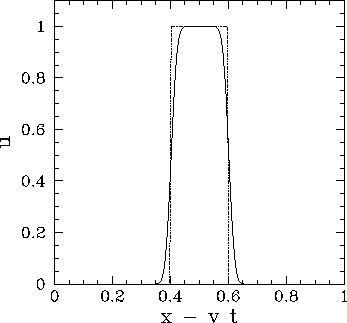Incidentally, it is easily demonstrated that the downwind differencing scheme,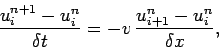(252)

is unconditionally unstable.Next: The 1-d wave equation Up: The wave equation Previous: The Crank-Nicholson scheme
Richard Fitzpatrick 2006-03-29